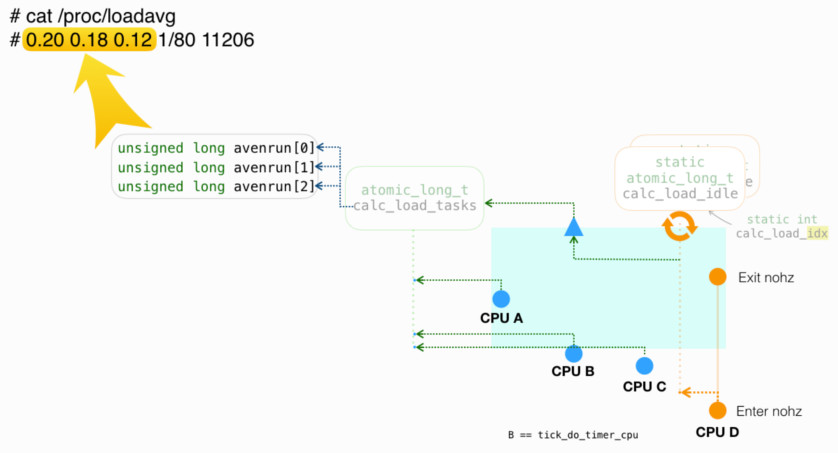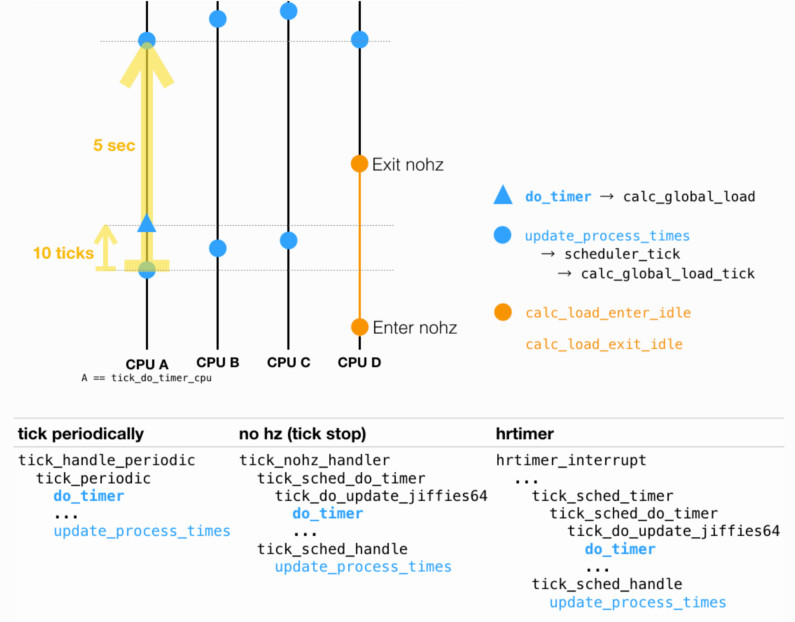微信公众号 关注我们 知识星球关注 @泰晓科技与数百位一线 Linux 工程师做朋友，您准备好了吗？周一到周五，天天有新文。 529 页合集等着领！# “茴”字有几种写法：系统负载是怎样计算的？（一）

 微信公众号 知识星球关注 @泰晓科技与数百位一线 Linux 工程师做朋友，您准备好了吗？周一到周五，天天有新文。 529 页合集等着领！

By Chen Jie of TinyLab.org 2017-06-25 00:25:17

## 前言

``````# cat /proc/loadavg
# 0.20 0.18 0.12 1/80 11206
``````
• 前三个数代表过去 1 / 5 / 15 分钟内，所有处于 就绪（等待和使用 CPU 资源）和 不可中断睡眠（等待和使用 IO 资源）的平均任务数，即平均负载。
• “1/80” 表示当前 1 个 任务就绪，总共 80 个任务。
• “11206” 代表最近一次分配出的 process ID 是 11206。

1. 如何用 int 类型来编码 一定精度的小数
2. 如何 printf 如上编码的小数？
3. 若要保留 n 位，如何四舍五入？## 统计量 avenrun``````/* 每 5 秒采样一次，1 分钟内的均值 */
float avenrun_f = avenrun_f * e^(-5/60) + calc_load_tasks * (1.0 - e^(-5/60);

/* 每 5 秒采样一次，5 分钟内的均值 */
float avenrun_f = avenrun_f * e^(-5/300) + calc_load_tasks * (1.0 - e^(-5/300);

/* 每 5 秒采样一次，15 分钟内的均值 */
float avenrun_f = avenrun_f * e^(-5/900) + calc_load_tasks * (1.0 - e^(-5/900);
``````

• Commit: 每隔 5s，各个 CPU 以 delta 形式，上报给 calc_load_tasks
• 每隔 5s 是通过在 tick 中比较 jiffies 和 `rq->calc_load_update` 做到的
• 下图例中，对应蓝色圆点
• Sampling: 每隔 5s，索引为 tick_do_timer_cpu 的 CPU 将 calc_load_tasks 累计入 avenrun
• 每隔 5s 是通过在 tick 中比较 jiffies 和 `calc_load_update` 做到的
• 下图例中，对应蓝色三角## 拾遗：针对 nohz 的补丁

• Patch commit：当前 CPU 进入 nohz，将其原本要提交的 delta，存入 calc_load_idle[idx]
• calc_load_idle 是个 “double buffer”：“front buffer” 由 calc_load_idx 所指出。
• 在 calc_global_load() → calc_load_fold_idle() 中，“front buffer” 被消费（“front buffer” 清 0）
• 在 calc_global_load() → calc_global_nohz() 中，swap “front/back buffer”。
• 退出 nohz 对应代码：calc_load_exit_idle() —— 主要是更新 `rq->calc_load_update`：取决于是否在 “10 ticks 的 sampling window” 内，或为 `calc_load_update + 5s`，或就是全局时间戳 `calc_load_update`
• Patch sampling：当全部 CPU 进入 nohz，采样间隔可能 >5s，即可能错过多个采样周期，需要一次性补回来：

``````/* code snippet in calc_global_nohz() */
if (!time_before(jiffies, sample_window + 10)) {
/*
* Catch-up, fold however many we are behind still
*/
delta = jiffies - sample_window - 10;
n = 1 + (delta / LOAD_FREQ);

active = active > 0 ? active * FIXED_1 : 0;

avenrun = calc_load_n(avenrun, EXP_1, active, n);
avenrun = calc_load_n(avenrun, EXP_5, active, n);
avenrun = calc_load_n(avenrun, EXP_15, active, n);

}
``````

``````/*
* a1 = a0 * e + a * (1 - e)
*
* a2 = a1 * e + a * (1 - e)
*    = (a0 * e + a * (1 - e)) * e + a * (1 - e)
*    = a0 * e^2 + a * (1 - e) * (1 + e)
*
* a3 = a2 * e + a * (1 - e)
*    = (a0 * e^2 + a * (1 - e) * (1 + e)) * e + a * (1 - e)
*    = a0 * e^3 + a * (1 - e) * (1 + e + e^2)
*
*  ...
*
* an = a0 * e^n + a * (1 - e) * (1 + e + ... + e^n-1) 
*    = a0 * e^n + a * (1 - e) * (1 - e^n)/(1 - e)
*    = a0 * e^n + a * (1 - e^n)
*
*  application of the geometric series:
*
*              n         1 - x^(n+1)
*     S_n := \Sum x^i = -------------
*             i=0          1 - x
*/
static unsigned long
unsigned long active, unsigned int n)
{
}
``````

``````/* 计算 x^n 的值 */
static unsigned long
fixed_power_int(unsigned long x, unsigned int n)
{
unsigned long result = FIXED_1;

if (n) {
for (;;) {
if (n & 1) {
/*
* 模拟浮点乘法，例如：
*  result = 0.21 * FIXED_1
*  x = 0.92 * FIXED_1
*  result * x = 0.21 * 0.92 * FIXED_1
*             = 0.21 FIXED_1 * 0.92 * FIXED_1 / FIXED_1
*/
result *= x;
result += FIXED_1 / 2; /* 四舍五入 */
result /= FIXED_1;
}
n >>= 1;
if (!n)
break;
x *= x;
x += FIXED_1 / 2; /* 四舍五入 */
x /= FIXED_1;
}
}

return result;
}
``````